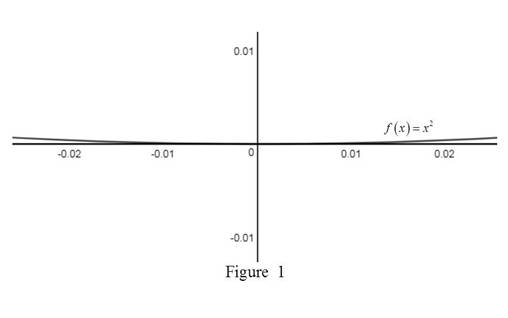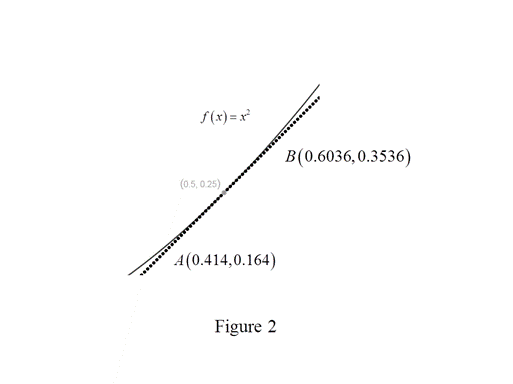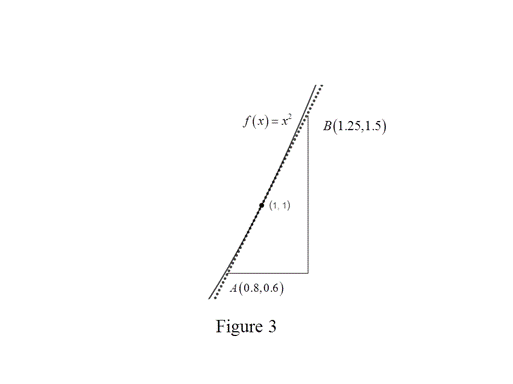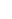# To estimate: The value of f ′ ( 0 ) , f ′ ( 1 2 ) , f ′ ( 1 ) and f ′ ( 2 ) by using a graphing device to zoom in on the graph of f.### Single Variable Calculus: Concepts...

4th Edition
James Stewart
Publisher: Cengage Learning
ISBN: 9781337687805### Single Variable Calculus: Concepts...

4th Edition
James Stewart
Publisher: Cengage Learning
ISBN: 9781337687805

#### Solutions

Chapter 2.7, Problem 17E

(a)

To determine

## To estimate: The value of f′(0), f′(12), f′(1) and f′(2) by using a graphing device to zoom in on the graph of f.

Expert Solution

The value of f(0), f(12), f(1) and f(2) are 0, 1, 2 and 4 respectively.

### Explanation of Solution

Given:

The function is f(x)=x2.

Estimation:

Obtain the value of f(x) at the point 0 as follows.

Use the online graphing calculator to zoom toward the point (0,0) as shown below in Figure 1.The calculation of f(0) is as follows,

From Figure 1, the tangent to the curve at x=0 is the x-axis.

Thus, f(0)=0.

Obtain the value of f(x) at the point 12.

Use the online graphing calculator to zoom toward the point (12,14) as shown below in Figure 2.The calculation of f(12) is as follows.

From Figure 2, the slope of the tangent line to the curve is computed as follows.

m=0.35360.1640.60360.4141

Thus, f(12)=1.

Obtain the value of f(x) at the point 1.

Use the online graphing calculator to zoom toward the point (1,1) as shown below in Figure 3.The calculation of f(1) is as follows.

From Figure 3, the slope of the tangent line to the curve is computed as follows.

m=1.50.61.250.8=0.90.45=2

Thus, f(1)=2.

Obtain f(x) at the point 2.

Use the online graphing calculator to zoom toward the point (2,4) as shown below in Figure 4.The calculation of f(2) is as follows.

From Figure 4, the slope of the tangent line is computed as follows.

m=251.52.25=30.75=334=4

Thus, f(2)=4.

(b)

To determine

### To deduce: The values of f′(−12),f′(−1) and f′(−2) using symmetry.

Expert Solution

The values of f(12),f(1) and f(2) are 1,2 and 4 respectively.

### Explanation of Solution

Result Used:

For an odd function f, f(x)=f(x).

For an even function f, f(x)=f(x).

Calculation:

The function f(x)=x2 is an even function.

Thus, the function f(x) is an odd function.

From part (a), f(12)=1,f(1)=2 and f(2)=4.

Using the symmetry,

f(12)=f(12)=1

Thus, f(12)=1.

Using the symmetry,

f(1)=f(1)=2

Thus, f(1)=2.

Using the symmetry,

f(2)=f(2)=4

Thus, f(2)=4.

Therefore, the values of f(12),f(1) and f(2) are 1,2 and 4 respectively.

(c)

To determine

### To guess: The formula for f′ by using part (a) and part (b).

Expert Solution

The formula of f is f(x)=2x

### Explanation of Solution

From part (a) and part (b),

f(12)=1=2(12)f(1)=2=2(1)

f(2)=4=2(2)

From the above calculations, it is observed that the derivative of the function is twice the input value.

Thus, f(x)=2x.

Thus, the formula of f is f(x)=2x.

(d)

To determine

Expert Solution

### Explanation of Solution

Definition used:

The derivation of a function is given by the formula

f(x)=limh0f(x+h)f(x)h

Proof:

Consider the function f(x)=x2.

Use the definition of derivative to obtain the derivative of f(x)=x2 as follows.

f(x)=limh0f(x+h)f(x)h=limh0(x+h)2(x)2h=limh0x2+h2+2xhx2h

Simplify the terms in numerator,

f(x)=limh0h2+2xhh=limh0h(h+2x)h

Since the limit h approaches zero but is not equal to zero, cancel the common term h from both the numerator and the denominator.

f(x)=limh0(h+2x)=limh0(h)+limh0(2x)=0+2xlimh0(1)=2x

So, f(x)=2x

Thus, the guess in part (c) is correct.

### Have a homework question?

Subscribe to bartleby learn! Ask subject matter experts 30 homework questions each month. Plus, you’ll have access to millions of step-by-step textbook answers!